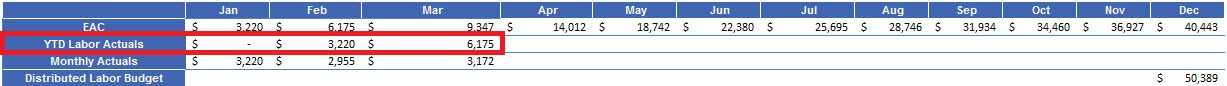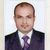# QlikView App Dev

Discussion Board for collaboration related to QlikView App Development.

Announcements
Join this live chat April 6, 10AM EST - QlikView to Qlik Sense REGISTER
cancel
Showing results for
Search instead for
Did you mean:Contributor

## YTD Calculation

Hi,

I want to calculate the YTD values and populate in a combo chart.

Find below the image which is the calculation in a spreadsheet.

Jan -  NIL

Feb - Monthly Actuals(Jan) - \$3,220

Mar - Monthly Actuals(Jan+Feb) - (\$3,200+2,995).=\$6,175..

Montly Actuals are calculated with the dimensions. Now the issue is with the YTD Labor Actuals.

Please suggest an idea to pull the values from Feb month in Qlikview expression as per the below snapshot. Thanks.3 RepliesMVP

For YTD calculation use like below

Eg:-

=Sum({\$<YourDatefield = {'>\$(=Max((YearStart(Today())))) <=\$(=Max(today()))'}>}  Value)Contributor
Author

I didnt get the correct result for the above set analysis.

The spreadsheet calculation is different from the actual YTD formula.

Can you please explain in brief, if the above set analysis can be applied for my case.Champion

Request you to refer master calender SIMPLE MASTER CALENDER , Please implement the same in your data model and give following formula for calculating YTD.

SUM({\$<FinancialYear=,FiscalYear={\$(=max(FiscalYear))},MonthName=,Quarter=

,[Posting Date]={">=\$(=YearStart(max({\$<FiscalYear={\$(=max(FiscalYear))}>}[Posting Date]),0,4))<=\$(=max({\$<FiscalYear={\$(=max(FiscalYear))}>}[Posting Date]))"}

>}[Sales Amount])

Hope this help you to calculate ytd.

Vikas